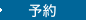•Operator Analysis : Holomorphic Functions as Functions of an Operator Variable (Cambridge Tracts in Mathematics)

• ただいまウェブストアではご注文を受け付けておりません。 ⇒古書を探す
• 製本 Hardcover:ハードカバー版／ページ数 280 p.
• 言語 ENG
• 商品コード 9781108485449
• DDC分類 512

Full Description

This book shows how operator theory interacts with function theory in one and several variables. The authors develop the theory in detail, leading the reader to the cutting edge of contemporary research. It starts with a treatment of the theory of bounded holomorphic functions on the unit disc. Model theory and the network realization formula are used to solve Nevanlinna-Pick interpolation problems, and the same techniques are shown to work on the bidisc, the symmetrized bidisc, and other domains. The techniques are powerful enough to prove the Julia-Caratheodory theorem on the bidisc, Lempert's theorem on invariant metrics in convex domains, the Oka extension theorem, and to generalize Loewner's matrix monotonicity results to several variables. In Part II, the book gives an introduction to non-commutative function theory, and shows how model theory and the network realization formula can be used to understand functions of non-commuting matrices.

Contents

Part I. Commutative Theoryapproaches to function theory; 2. Operator analysis on D: model formulas, lurking Isometries, and positivity arguments; 3. Further development of models on the disc; 4. Operator analysis on D2; 5. Caratheodory-Julia theory on the disc and the bidisc; 6. Herglotz and Nevanlinna representations in several variables; 7. Model theory on the symmetrized bidisc; 8. Spectral sets: three case studies; 9. Calcular norms; 10. Operator monotone functions; Part II. Non-Commutative Theory: 11. Motivation for non-commutative functions; 12. Basic properties of non-commutative functions; 13. Montel theorems; 14. Free holomorphic functions; 15. The implicit function theorem; 16. Noncommutative functional calculus; Notation.